# RS Aggarwal Class 9 Solutions Chapter 2 Polynomials Ex 2K

## RS Aggarwal Class 9 Solutions Chapter 2 Polynomials Ex 2K

These Solutions are part of RS Aggarwal Solutions Class 9. Here we have given RS Aggarwal Solutions Class 9 Chapter 2 Polynomials Ex 2K.

Other Exercises

Question 1.
Solution:
125a3 + b3 + 64c3 – 60abc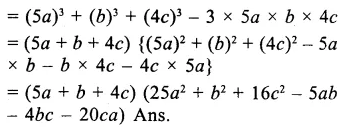Question 2.
Solution:
a3 + 8b3 + 64c3 – 24abc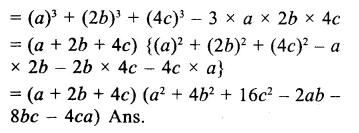Question 3.
Solution:
1 + b3 + 8c3 – 6bc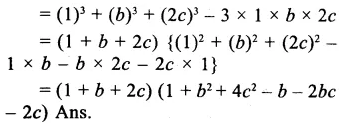Question 4.
Solution:
216 + 27b3 + 8c3 – 108bc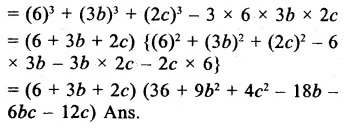Question 5.
Solution:
27a3 – b3 + 8c3 + 18abc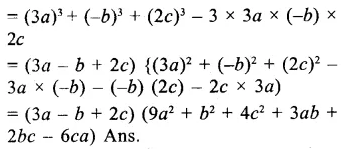Question 6.
Solution:
8a3 + 125b3 – 64c3 + 120abc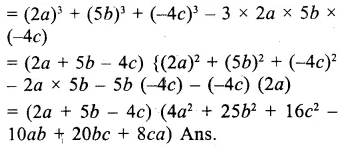Question 7.
Solution:
8 – 27b3 – 343c3 – 126bc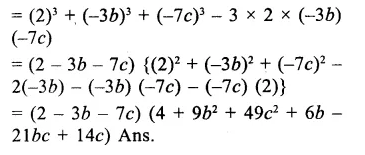Question 8.
Solution:
125 – 8x3 – 27y3 – 90xy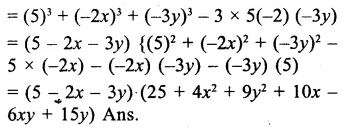Question 9.
Solution:
2√2a3 + 16√2b3 + c3 – 12abc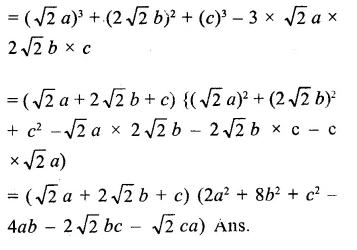Question 10.
Solution:
x3 + y3 – 12xy + 64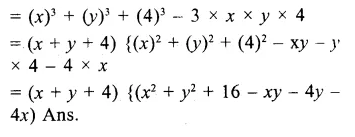Question 11.
Solution:
(a – b)3 + (b – c)3 + (c – a)3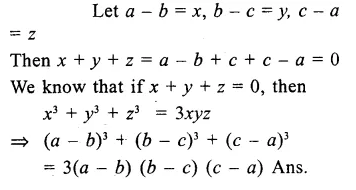Question 12.
Solution:
(3a – 2b)3 + (26 – 5c)3 + (5c – 3a)3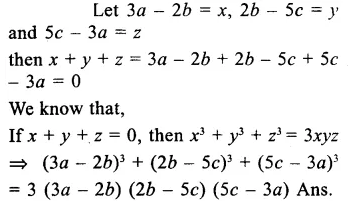Question 13.
Solution:
a3 (b – c)3 + b3 (c – a)3 + c3 (a – b)3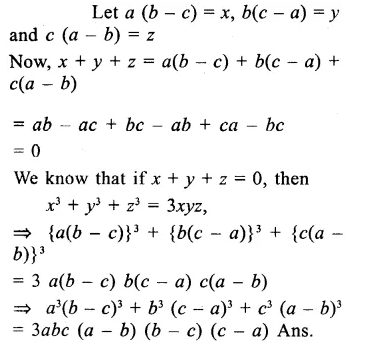Question 14.
Solution:
(5a – 7b)3 + (9c – 5a)3 + (7b – 9c)3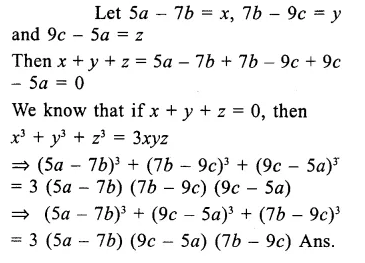Question 15.
Solution:
(x + y – z) (x2 + y2 + z2 – xy + yz + zx)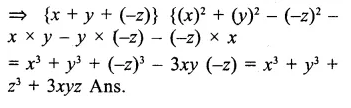Question 16.
Solution:
(x – 2y + 3) (x2 + 4y2 + 2xy -3x + 6y + 9)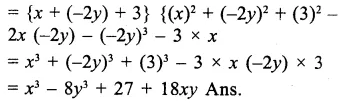Question 17.
Solution:
(x – 2y – z) (x2 + 4y2 + z2 + 2xy + zx- 2yz)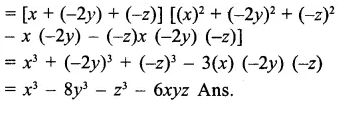Question 18.
Solution:
x + y + 4 = 0,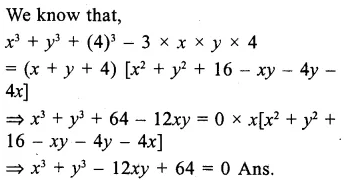Question 19.
Solution:
x = 2y + 6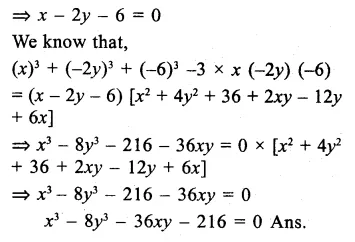Hope given RS Aggarwal Solutions Class 9 Chapter 2 Polynomials Ex 2K are helpful to complete your math homework.

If you have any doubts, please comment below. Learn Insta try to provide online math tutoring for you.